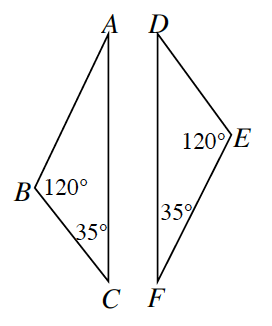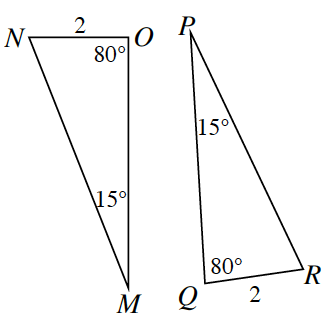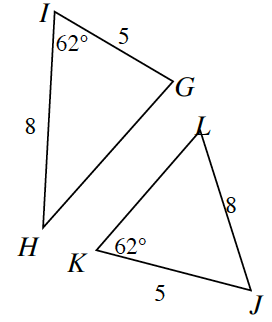### Home > INT2 > Chapter 3 > Lesson 3.2.3 > Problem3-95

3-95.

For each pair of triangles below, determine if the triangles are congruent, similar but not congruent, or if their relationship cannot be determined from the information given. If they are congruent, organize your reasoning into a flowchart. Remember that the triangles may not be drawn to scale.

Use the triangle congruence conditions.

1.Similar by $\text{AA}$ ~.

1.$ΔMON≅ΔPQR$
$AAS ≅$ or $ASA ≅$

1.Are the corresponding parts in the correct places?